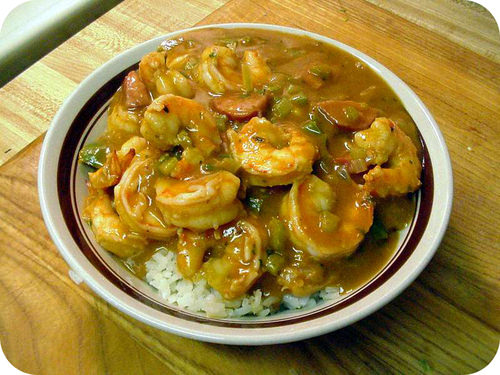# 11.2: Chemical Equations

$$\newcommand{\vecs}{\overset { \rightharpoonup} {\mathbf{#1}} }$$ $$\newcommand{\vecd}{\overset{-\!-\!\rightharpoonup}{\vphantom{a}\smash {#1}}}$$$$\newcommand{\id}{\mathrm{id}}$$ $$\newcommand{\Span}{\mathrm{span}}$$ $$\newcommand{\kernel}{\mathrm{null}\,}$$ $$\newcommand{\range}{\mathrm{range}\,}$$ $$\newcommand{\RealPart}{\mathrm{Re}}$$ $$\newcommand{\ImaginaryPart}{\mathrm{Im}}$$ $$\newcommand{\Argument}{\mathrm{Arg}}$$ $$\newcommand{\norm}{\| #1 \|}$$ $$\newcommand{\inner}{\langle #1, #2 \rangle}$$ $$\newcommand{\Span}{\mathrm{span}}$$ $$\newcommand{\id}{\mathrm{id}}$$ $$\newcommand{\Span}{\mathrm{span}}$$ $$\newcommand{\kernel}{\mathrm{null}\,}$$ $$\newcommand{\range}{\mathrm{range}\,}$$ $$\newcommand{\RealPart}{\mathrm{Re}}$$ $$\newcommand{\ImaginaryPart}{\mathrm{Im}}$$ $$\newcommand{\Argument}{\mathrm{Arg}}$$ $$\newcommand{\norm}{\| #1 \|}$$ $$\newcommand{\inner}{\langle #1, #2 \rangle}$$ $$\newcommand{\Span}{\mathrm{span}}$$Figure $$\PageIndex{1}$$ (Credit: jons2 at pdphoto.org; Source: http://commons.wikimedia.org/wiki/File:Shrimp_gumbo.jpg; License: Public Domain)

### How do you make shrimp gumbo?

Shrimp gumbo is one of many dishes that are part of the Cajun culture in Louisiana. It's a spicy dish that needs careful control of all the ingredients so that it has a "kick", but is not overwhelming. Recipes convey not only what the preparation entails, but also describe how much of each ingredient is needed, and the details of how to cook the meal. Similarly, we need this type of information in order to carry out chemical reactions successfully and safely.

## Chemical Equations

Word equations are time-consuming to write and do not prove to be convenient for many of the things that chemists need to do with equations. A chemical equation is a representation of a chemical reaction that displays the reactants and products with chemical formulas. The chemical equation for the reaction of methane with oxygen is shown:

$\ce{CH_4} + \ce{O_2} \rightarrow \ce{CO_2} + \ce{H_2O}\nonumber$

The equation above, called a skeleton equation, is an equation that shows only the formulas of the reactants and products with nothing to indicate the relative amounts. The first step in writing an accurate chemical equation is to write the skeleton equation, making sure that the formulas of all substances involved are written correctly. All reactants are written to the left of the yield arrow, separated from one another by a plus sign. Likewise, products are written to the right of the yield arrow, also separated with a plus sign.

It is often important to know the physical states of the reactants and products taking part in a reaction. To do this, put the appropriate symbol in parentheses after each formula: $$\left( s \right)$$ for solid, $$\left( l \right)$$ for liquid, $$\left( g \right)$$ for gas, and $$\left( aq \right)$$ for an aqueous (water-based) solution. The previous reaction becomes:

$\ce{CH_4} \left( g \right) + \ce{O_2} \left( g \right) \rightarrow \ce{CO_2} \left( g \right) + \ce{H_2O} \left( l \right)\nonumber$

The table below shows a listing of symbols used in chemical equations. Some, such as the double arrow which represents equilibrium, and the use of a catalyst in a reaction, will be detailed in other concepts.

Symbols Description
Table $$\PageIndex{1}$$: Symbols Used in Chemical Equations
$$+$$ Used to separate multiple reactants or products.
$$\rightarrow$$ Yield sign; separates reactants from products.
$$\rightleftharpoons$$ Replaces the yield sign for reversible reactions that reach equilibrium.
$$\left( s \right)$$ Reactant or product in the solid state.
$$\left( l \right)$$ Reactant or product in the liquid state.
$$\left( g \right)$$ Reactant or product in the gaseous state.
$$\left( aq \right)$$ Reactant or product in an aqueous solution (dissolved in water).
$$\overset{\ce{Pt}}{\rightarrow}$$ Formula written above the arrow is used as a catalyst in the reaction.
$$\overset{\Delta}{\rightarrow}$$ Triangle indicates that the reaction is being heated.

## Summary

• A chemical equation is representation a chemical reaction using chemical formulas reactants and products, as well as symbols.
• Symbols used in chemical equations are described and explained.

## Review

1. What does a skeleton equation tell you?
2. Why would you want to know the physical state of materials in a chemical reaction?
3. In a chemical reaction what does symbol → mean?
4. If I see Δ over the arrow, what does this indicate?

11.2: Chemical Equations is shared under a CK-12 license and was authored, remixed, and/or curated by CK-12 Foundation via source content that was edited to conform to the style and standards of the LibreTexts platform; a detailed edit history is available upon request.